# Solving a popular problem about books

The modern school curriculum is much different from the one I studied. At least, we are talking about mathematics, since it was this science that was and is central to me. Sometimes even in the 5th grade you can find math problems that even adults cannot solve. Especially if these tasks are taken from the Olympiad works. Sometimes parents of schoolchildren turn to me to help sort out this or that problem. Basically, these are tasks on logic and combinatorics.

In this article, I will analyze one of the simplest tasks – a popular task about books. There may be more than one solution, but my method may seem one of the most difficult. First, we formulate the problem in a general form, derive the solution formula, and then consider a particular case as an example. It is a special case that occurs in problems.

Available for sale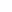books. The cost of all books except the first is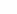rubles, the cost of all books, except for the second, isruble and so on, the cost of all books except the last one (-oh) is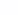rubles. How much does each book cost individually?

### Solution

Denote by variablespriceth book, where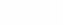. That is, the cost of the first book –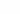the cost of the second book –and so on, the cost of the last book –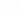. In order not to fence large formulas, for clarity, we denote the variable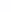the cost of all books, that is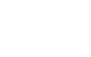According to the task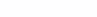,and so on,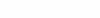. These expressions can be written in the system of equations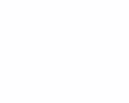Adding the left and right parts of the system of equations, we obtain the following equality: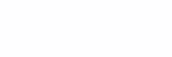Let’s expand the brackets on the left side of the equation.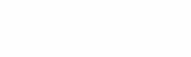We see that the first term on the left side of the equality (reduced) is nothing but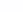, since the content under the sum sign is independent of the summation index. And the second term (subtracted) is equal to, given the notation at the beginning. Therefore it turns out: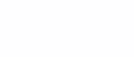Obviously you can takefor the bracket.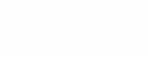From this we obtain an expression forthat is, we find the cost of all books.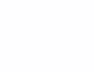According to the condition of the problem, the costs of all books are known, except for each (values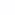). So to find the cost of each bookyou need from the cost of all bookssubtract: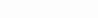or, finally: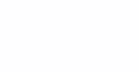where.

As an example, consider a special case of this problem for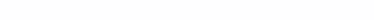The formulation of this problem and options for solving it can be easily found on the Internet in numerous forums. This problem is found in the 4th grade mathematics textbook. Substitute these values ​​into the final formula. First, find the cost of all books: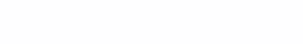Then we find the cost of each book: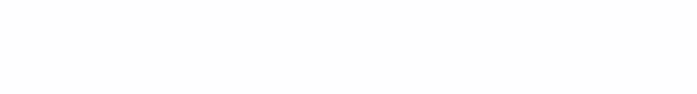Answer: 16, 14, 12, 10 rubles.